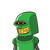# Which expression is equivalent to 55 + 2x + 5? * 3 points 2(25 + x) + 5 30(2 + x) 60(1 + x) 2(30 + x

Which expression is equivalent to 55 + 2x + 5? *
3 points
2(25 + x) + 5
30(2 + x)
60(1 + x)
2(30 + x)

### 1 thought on “Which expression is equivalent to 55 + 2x + 5? *<br /> 3 points<br /> 2(25 + x) + 5<br /> 30(2 + x)<br /> 60(1 + x)<br /> 2(30 + x”

1.part d

Step-by-step explanation:

2(25 + x ) + 5

50 + 2x + 5

2x+ 55

part B

60+30x

part c

60+ 60x

part d

60+ 2x

55+ 2x+5